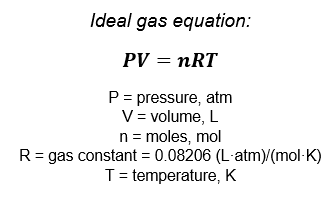# Problem: Magnesium can be used as a "getter" in evacuated enclosures to react with the last traces of oxygen. (The magnesium is usually heated by passing an electric current through a wire or ribbon of the metal.) If an enclosure of 0.462 L has a partial pressure of O2 of 3.6 × 10−6 torr at 28oC, what mass of magnesium will react according to the following equation: 2 Mg(s) + O2(g) → 2 MgO(s)?

###### FREE Expert Solution

First, calculate how many moles of O2(g) is present in the enclosure using the ideal gas equation. Then do a mole to mole comparison to determine the amount of Mg(s) that will react with O2(g):Given:###### Problem Details

Magnesium can be used as a "getter" in evacuated enclosures to react with the last traces of oxygen. (The magnesium is usually heated by passing an electric current through a wire or ribbon of the metal.) If an enclosure of 0.462 L has a partial pressure of O2 of 3.6 × 10−6 torr at 28oC, what mass of magnesium will react according to the following equation: 2 Mg(s) + O2(g) → 2 MgO(s)?